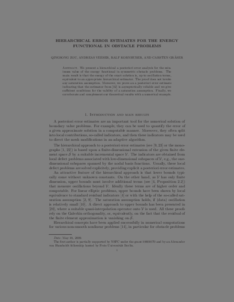Repository: Freie Universität Berlin, Math Department

# Hierarchical error estimates for the energy functional in obstacle problems

Zou, Q. and Veeser, A. and Kornhuber, R. and Gräser, C. (2011) Hierarchical error estimates for the energy functional in obstacle problems. Numerische Mathematik, 117 (4). pp. 653-677. ISSN 0029-599XPreview

203kB

Official URL: http://dx.doi.org/10.1007/s00211-011-0364-5

## Abstract

We present a hierarchical a posteriori error analysis for the minimum value of the energy functional in symmetric obstacle problems. The main result is that the error in the energy minimum is, up to oscillation terms, equivalent to an appropriate hierarchical estimator. The proof does not invoke any saturation assumption. We even show that small oscillation implies a related saturation assumption. In addition, we prove efficiency and reliability of an a posteriori estimate of the discretization error and thereby cast some light on the theoretical understanding of previous hierarchical estimators. Finally, we illustrate our theoretical results by numerical computations.

Item Type: Article Mathematical and Computer Sciences > Mathematics > Numerical Analysis Department of Mathematics and Computer Science > Institute of Mathematics 1800 Ekaterina Engel 18 Feb 2016 09:58 03 Mar 2017 14:41

Repository Staff Only: item control page